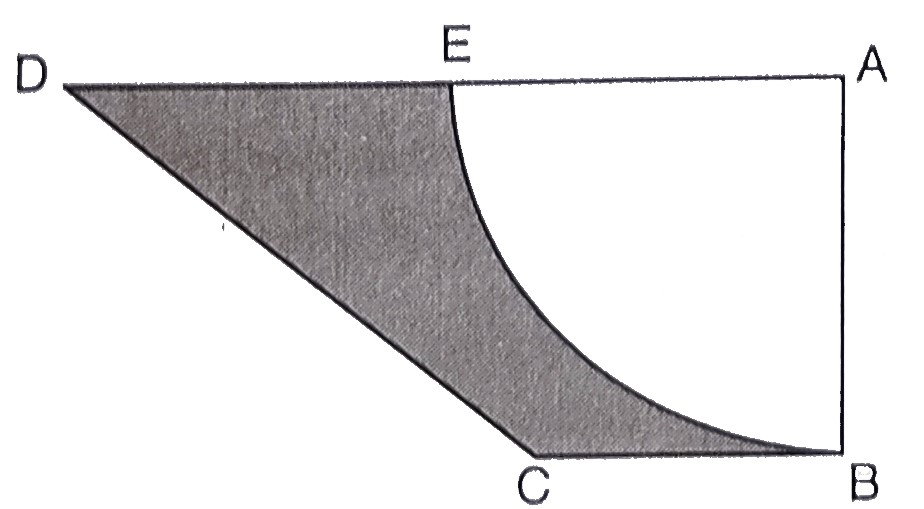"
">

# In the below figure, $A B C D$ is a trapezium of area $24.5 \mathrm{~cm}^{2} .$ In it, $A D \| B C, \angle D A B=90^{\circ}$, $A D=10 \mathrm{~cm}$ and $B C=4 \mathrm{~cm}$. If $A B E$ is a quadrant of a circle, find the area of the shaded region. (Take $\pi=22 / 7)$."

Given:

$A B C D$ is a trapezium of area $24.5 \mathrm{~cm}^{2} .$

$A D \| B C, \angle D A B=90^{\circ}$, $A D=10 \mathrm{~cm}$ and $B C=4 \mathrm{~cm}$.

$A B E$ is a quadrant of a circle.

To do:

We have to find the area of the shaded region.

Solution:

Area of trapezium $ABCD =\frac{1}{2}(\mathrm{AD}+\mathrm{BC}) \times \mathrm{AB}$

$24.5=\frac{1}{2}(10+4) h$

$24.5=7h$

$h=\frac{24.5}{7}$

$h=3.5 \mathrm{~cm}$

This implies,

Radius of the quadrant $=3.5$

$=\frac{7}{2} \mathrm{~cm}$

This implies,

Area of quadrant $=\frac{1}{4} \pi r^{2}$

$=\frac{1}{4} \times \frac{22}{7} \times (\frac{7}{2})^2$

$=\frac{77}{8}$

$=9.625 \mathrm{~cm}^{2}$

Therefore,

Area of the shaded region $=24.5-9.625$

$=24.500-9.625$

$=14.875 \mathrm{~cm}^{2}$

The area of the shaded region is $14.875\ cm^2$.

Updated on: 10-Oct-2022

29 Views